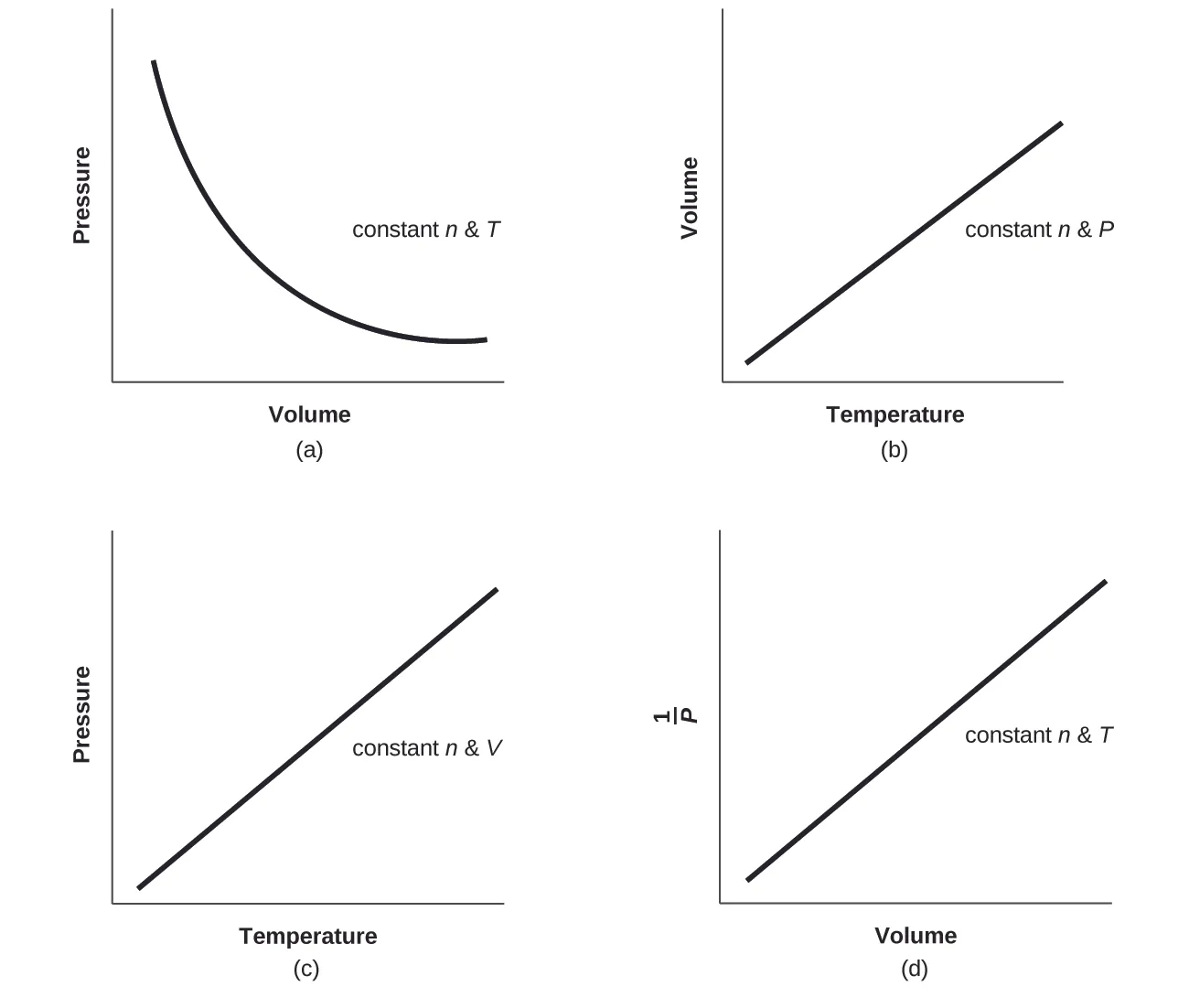Chemistry

# Chapter 9

ChemistryChapter 9

1.

The cutting edge of a knife that has been sharpened has a smaller surface area than a dull knife. Since pressure is force per unit area, a sharp knife will exert a higher pressure with the same amount of force and cut through material more effectively.

3.

Lying down distributes your weight over a larger surface area, exerting less pressure on the ice compared to standing up. If you exert less pressure, you are less likely to break through thin ice.

5.

0.809 atm; 82.0 kPa

7.

2.2 $××$ 102 kPa

9.

Earth: 14.7 lb in–2; Venus: 1.31 × 103 lb in−2

11.

(a) 101.5 kPa; (b) 51 torr drop

13.

(a) 264 torr; (b) 35,200 Pa; (c) 0.352 bar

15.

(a) 623 mm Hg; (b) 0.820 atm; (c) 83.1 kPa

17.

With a closed-end manometer, no change would be observed, since the vaporized liquid would contribute equal, opposing pressures in both arms of the manometer tube. However, with an open-ended manometer, a higher pressure reading of the gas would be obtained than expected, since Pgas = Patm + Pvol liquid.

19.

As the bubbles rise, the pressure decreases, so their volume increases as suggested by Boyle’s law.

21.

(a) The number of particles in the gas increases as the volume increases. (b) temperature, pressure

23.

The curve would be farther to the right and higher up, but the same basic shape.

25.

27.

3.40 $××$ 103 torr

29.

12.1 L

31.

217 L

33.

8.190 $××$ 10–2 mol; 5.553 g

35.

(a) 7.24 $××$ 10–2 g; (b) 23.1 g; (c) 1.5 $××$ 10–4 g

37.

5561 L

39.

46.4 g

41.

For a gas exhibiting ideal behavior:43.

(a) 1.85 L CCl2F2; (b) 4.66 L CH3CH2F

45.

0.644 atm

47.

The pressure decreases by a factor of 3.

49.

4.64 g L−1

51.

38.8 g

53.

72.0 g mol−1

55.

88.1 g mol−1; PF3

57.

141 atm, 107,000 torr, 14,300 kPa

59.

CH4: 276 kPa; C2H6: 27 kPa; C3H8: 3.4 kPa

61.

Yes

63.

740 torr

65.

(a) Determine the moles of HgO that decompose; using the chemical equation, determine the moles of O2 produced by decomposition of this amount of HgO; and determine the volume of O2 from the moles of O2, temperature, and pressure. (b) 0.308 L

67.

(a) Determine the molar mass of CCl2F2. From the balanced equation, calculate the moles of H2 needed for the complete reaction. From the ideal gas law, convert moles of H2 into volume. (b) 3.72 $××$ 103 L

69.

(a) Balance the equation. Determine the grams of CO2 produced and the number of moles. From the ideal gas law, determine the volume of gas. (b) 7.43 $××$ 105 L

71.

42.00 L

73.

(a) 18.0 L; (b) 0.533 atm

75.

10.57 L O2

77.

5.40 $××$ 105 L

79.

XeF2

81.

4.2 hours

83.

Effusion can be defined as the process by which a gas escapes through a pinhole into a vacuum. Graham’s law states that with a mixture of two gases A and B: $(rate Arate B)=(molar mass of Bmolar mass of A)1/2.(rate Arate B)=(molar mass of Bmolar mass of A)1/2.$ Both A and B are in the same container at the same temperature, and therefore will have the same kinetic energy:
$KEA=KEB KE=12mv2KEA=KEB KE=12mv2$
Therefore, $12mAvA2=12mBvB212mAvA2=12mBvB2$
$vA2vB2=mBmAvA2vB2=mBmA$
$(vA2vB2)1/2=(mBmA)1/2(vA2vB2)1/2=(mBmA)1/2$
$vAvB=(mBmA)1/2vAvB=(mBmA)1/2$

85.

F2, N2O, Cl2, H2S

87.

1.4; 1.2

89.

51.7 cm

91.

Yes. At any given instant, there are a range of values of molecular speeds in a sample of gas. Any single molecule can speed up or slow down as it collides with other molecules. The average velocity of all the molecules is constant at constant temperature.

93.

H2O. Cooling slows the velocities of the He atoms, causing them to behave as though they were heavier.

95.

(a) The number of collisions per unit area of the container wall is constant. (b) The average kinetic energy doubles. (c) The root mean square speed increases to $22$ times its initial value; urms is proportional to $KEavg.KEavg.$

97.

(a) equal; (b) less than; (c) 29.48 g mol−1; (d) 1.0966 g L−1; (e) 0.129 g/L; (f) 4.01 $××$ 105 g; net lifting capacity = 384 lb; (g) 270 L; (h) 39.1 kJ min−1

99.

Gases C, E, and F

101.

The gas behavior most like an ideal gas will occur under the conditions in (b). Molecules have high speeds and move through greater distances between collision; they also have shorter contact times and interactions are less likely. Deviations occur with the conditions described in (a) and (c). Under conditions of (a), some gases may liquefy. Under conditions of (c), most gases will liquefy.

103.

SF6

105.

(a) A straight horizontal line at 1.0; (b) When real gases are at low pressures and high temperatures they behave close enough to ideal gases that they are approximated as such, however, in some cases, we see that at a high pressure and temperature, the ideal gas approximation breaks down and is significantly different from the pressure calculated by the ideal gas equation (c) The greater the compressibility, the more the volume matters. At low pressures, the correction factor for intermolecular attractions is more significant, and the effect of the volume of the gas molecules on Z would be a small lowering compressibility. At higher pressures, the effect of the volume of the gas molecules themselves on Z would increase compressibility (see Figure 9.35) (d) Once again, at low pressures, the effect of intermolecular attractions on Z would be more important than the correction factor for the volume of the gas molecules themselves, though perhaps still small. At higher pressures and low temperatures, the effect of intermolecular attractions would be larger. See Figure 9.35. (e) low temperatures

Order a print copy

As an Amazon Associate we earn from qualifying purchases.